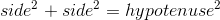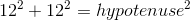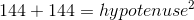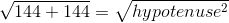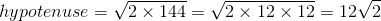# High School Math : How to find the length of the diagonal of a square

## Example Questions

### Example Question #1 : How To Find The Length Of The Diagonal Of A Square

What is the length of a diagonal of a square with a side length? Round to the nearest tenth.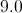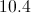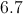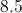Explanation:

A square is comprised of two 45-45-90 right triangles. The hypotenuse of a 45-45-90 right triangle follows the rule below, whereis the length of the sides.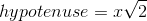In this instance,is equal to 6.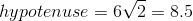### Example Question #2 : How To Find The Length Of The Diagonal Of A Square

A square has sides of. What is the length of the diagonal of this square?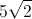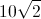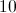Explanation:

To find the diagonal of the square, we effectively cut the square into two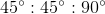triangles.

The pattern for the sides of ais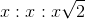.

Since two sides are equal to, this triangle will have sides of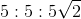.

Therefore, the diagonal (the hypotenuse) will have a length of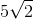.

### Example Question #3 : How To Find The Length Of The Diagonal Of A Square

A square has sides of. What is the length of the diagonal of this square?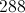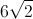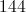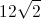Explanation:

To find the diagonal of the square, we effectively cut the square into twotriangles.

The pattern for the sides of ais.

Since two sides are equal to, this triangle will have sides of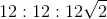.

Therefore, the diagonal (the hypotenuse) will have a length of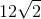.

### Example Question #4 : How To Find The Length Of The Diagonal Of A Square

What is the length of the diagonal of a square with a side length of?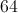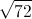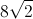Explanation:

To find the diagonal of a square, we must use the side length to create a 90 degree triangle with side lengths of, and a hypotenuse which is equal to the diagonal.

Pythagorean’s Theorem states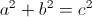, where a and b are the legs and c is the hypotenuse.

Takeandand plug them into the equation forand: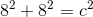After squaring the numbers, add them together: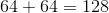Once you have the sum, take the square root of both sides: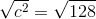Simplify to find the answer: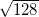, or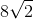.

### Example Question #1 : Squares

What is the length of the diagonal of a 7-by-7 square? (Round to the nearest tenth.)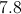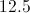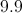Explanation:

To find the diagonal of a square we must use the side lengths to create a 90 degree triangle with side lengths of 7 and a hypotenuse which is equal to the diagonal.

We can use the Pythagorean Theorem here to solve for the hypotenuse of a right triangle.

The Pythagorean Theorem states, where a and b are the sidelengths and c is the hypotenuse.

Plug the side lengths into the equation asand: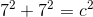Square the numbers: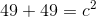Add the terms on the left side of the equation together: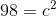Take the square root of both sides: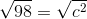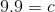Therefore the length of the diagonal is 9.9.

### Example Question #6 : How To Find The Length Of The Diagonal Of A Square

The perimeter of a square is 48. What is the length of its diagonal?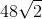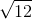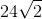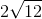Explanation:

Perimeter = side * 4

48 = side * 4

Side = 12

We can break up the square into two equal right triangles. The diagonal of the sqaure is then the hypotenuse of these two triangles.

Therefore, we can use the Pythagorean Theorem to solve for the diagonal: